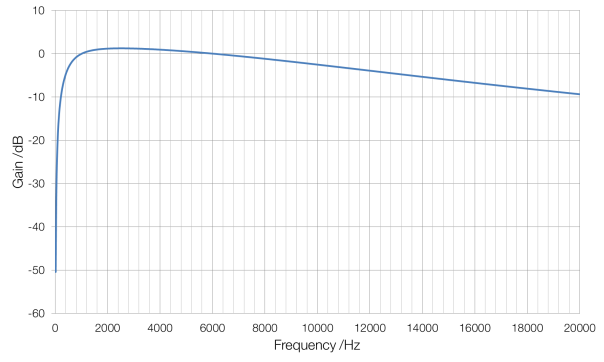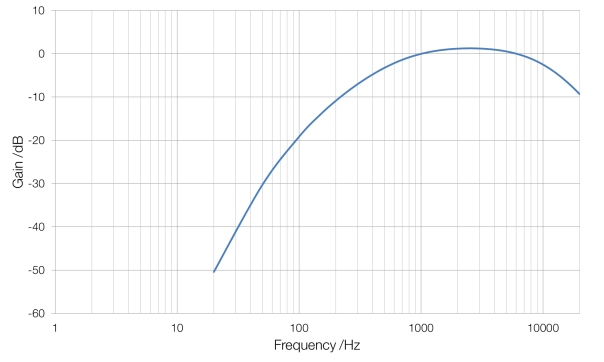# dBA and Grey Noise

The human ear doesn’t hear equally well at all frequencies. The ear is much less sensitive to low frequencies, below about 1000 Hz, and to high frequencies above about 6000 Hz, and peaks in sensitivity at around 2500 Hz.

A microphone doesn’t have the same issue. This means that after sound is recorded, a filter is applied so that the recorded sound mimics what a human ear would have heard. This filter is called A-weighting, and the volume of sound that is recorded is referred to as dB(A).dB(A) weighting (linear frequency scale)dB(A) weighting (logarithmic frequency scale)

White noise is often taken to be equally loud at all frequencies, but this is not the case: although the sound that is produced is equally loud at all frequencies, this is not what the ear hears. Grey noise is white noise that has been A-weighted so that it is heard to be equally loud at all frequencies.

White noise:

# The Fibonacci Sequence and Converting from Miles to Kilometres

The Fibonacci Sequence is a very famous sequence of numbers, named after Italian mathematician Leonardo Fibonacci (though the sequence had already been described by Indian mathematicians). Each term in the sequence is composed of the sum of the previous two terms:

F = 1, 1, 2, 3, 5, 8, 13, 21, 34, 55 and so on …

As the sequence grows longer the ratio of each term to the previous term becomes closer and closer to the golden ratio of 1.618 (to four significant figures). This is helpful because the ratio of kilometres to miles is 1.609, which differs by only 0.556 percent.

Therefore, to quickly convert from miles to kilometres you only need to find the value in miles in the Fibonacci sequence and look at the next number in the sequence: 21 miles is 34 kilometres, 34 miles is 55 kilometres and so on. If converting in the other direction, look at the previous term to convert from kilometres to miles.